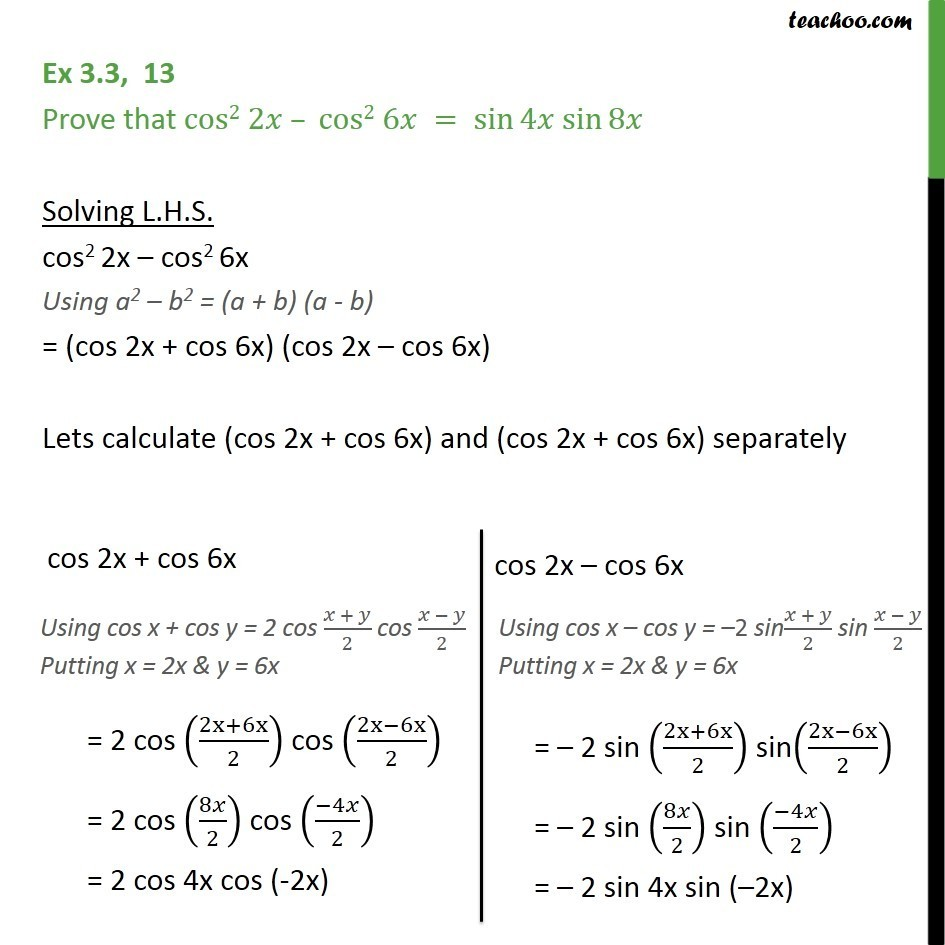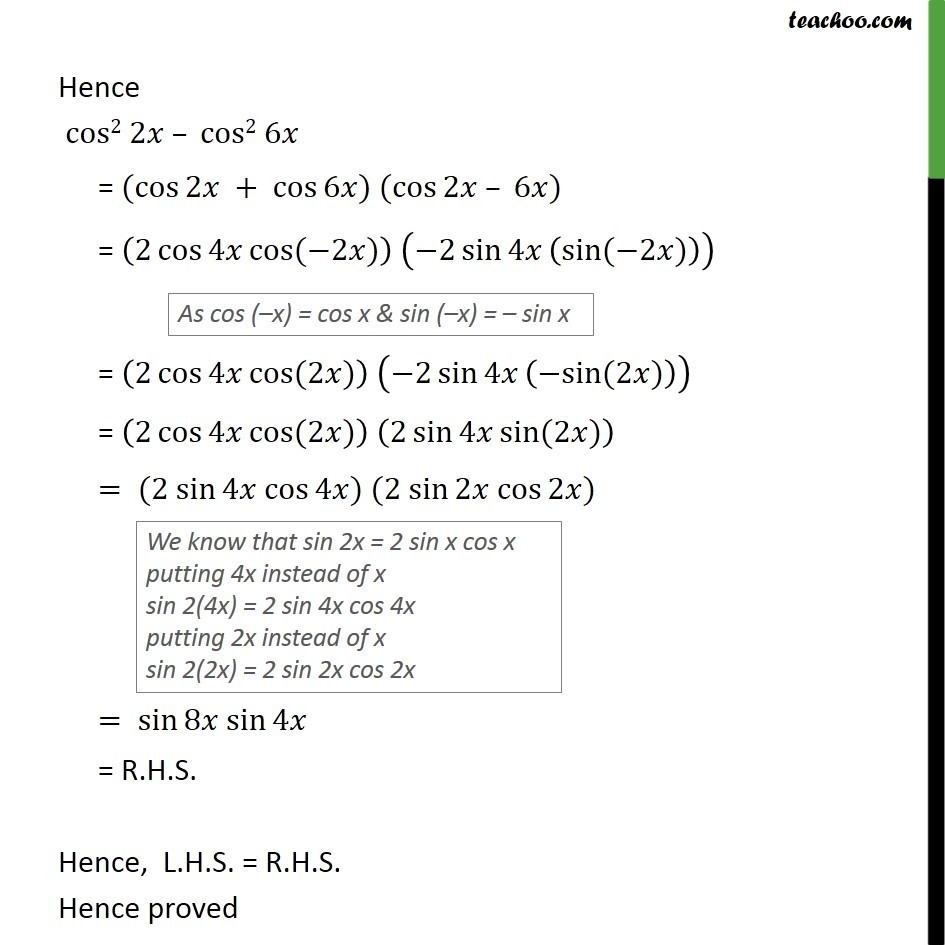1. Chapter 3 Class 11 Trigonometric Functions (Term 2)
2. Concept wise
3. (x + y) formula

Transcript

Ex 3.3, 13 Prove that cos2 2𝑥 – cos2 6𝑥 = sin⁡4𝑥 sin⁡8𝑥 Solving L.H.S. cos2 2x – cos2 6x Using a2 – b2 = (a + b) (a - b) = (cos 2x + cos 6x) (cos 2x – cos 6x) Lets calculate (cos 2x + cos 6x) and (cos 2x + cos 6x) separately Hence cos2 2𝑥 – cos2 6𝑥 = (cos⁡2𝑥 + cos⁡6𝑥) (cos⁡2𝑥 – 6𝑥) = (2 cos⁡〖4𝑥 cos⁡〖(−2𝑥)〗 〗 ) (−2 sin⁡4𝑥 (sin⁡〖(−2𝑥)〗 )) = (2 cos⁡〖4𝑥 cos⁡〖(2𝑥)〗 〗 ) (−2 sin⁡4𝑥 (〖−sin〗⁡〖(2𝑥)〗 )) = (2 cos⁡〖4𝑥 cos⁡〖(2𝑥)〗 〗 ) (2 sin⁡4𝑥 sin⁡〖(2𝑥)〗 ) = (2 sin⁡4𝑥 cos⁡4𝑥) (2 sin⁡2𝑥 cos⁡2𝑥) = sin⁡8𝑥 sin⁡4𝑥 = R.H.S. Hence, L.H.S. = R.H.S. Hence proved